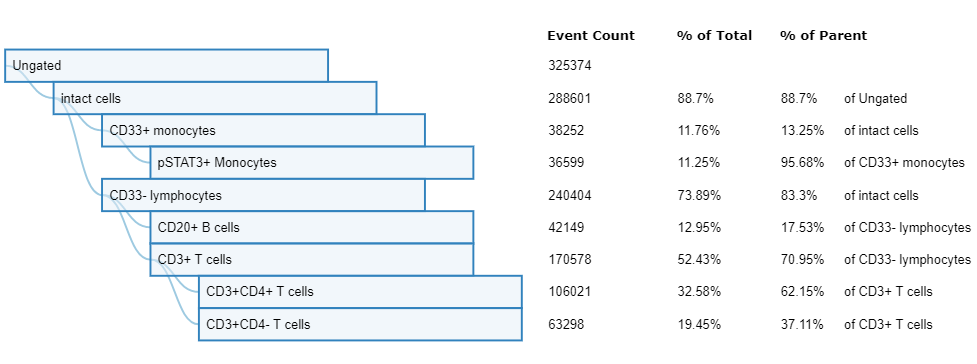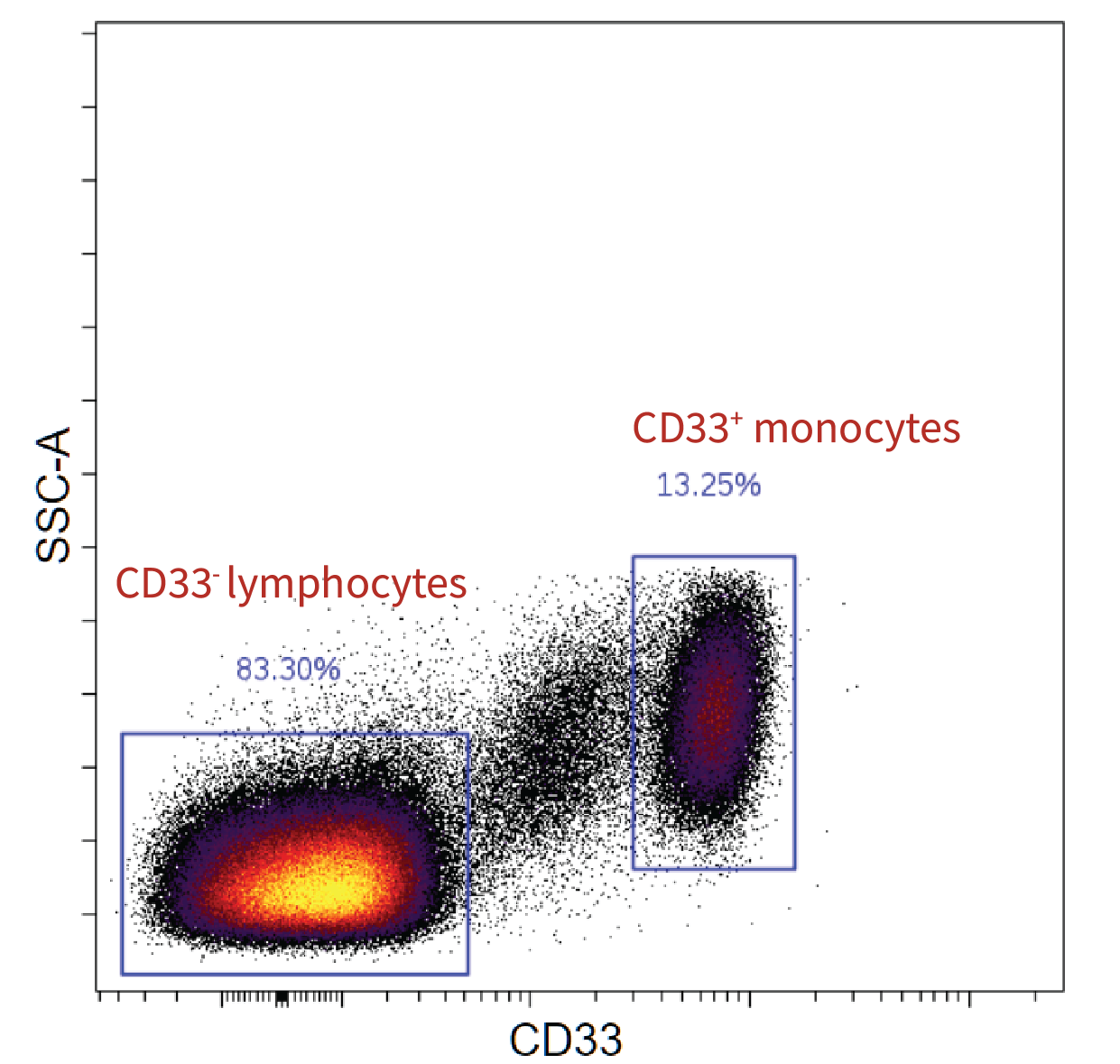# Statistics in Cytobank: Percent of Population, Percent in Gate, and Event Count Ratio

Background

Cytobank offers the percent of population and event count ratio statistics in the export statistics tool. The percent  in gate statistic is available  in the gating interface, export statistics tool, and Illustration Editor.  It is useful to understand exactly how each statistic is calculated to determine the appropriate statistic for your analysis. The first thing to remember is that in Cytobank, a population and a gate are separate entities. Reading more about the difference between a population and a gate will be useful to understand each of the following  statistics.

Percent of Population

To calculate percent of population, two populations are chosen, X and Y, and consideration is given to the hierarchical relationship between these two populations. The total number of events in population X that are also in population Y divided by the total number of events in population Y determines the percent of population. In other words, the math is as follows for the examples below:(gating hierarchy for  examples below)

Example 1):
Population X = CD3+ T cells
Population Y = Intact cells
Total events in X that are in Y: 170578
Total events in Y: 288601
Percent of population = (170578/288601)*100 = 59.1051%

Example 2):
Population X = CD33+ monocytes
Population Y = CD3+ T cells
Total events in X that are in Y: 0
Total events in Y: 170578
Percent of population = (0/170578)*100 = 0%

In this example, the percent of population is zero. This is because the total number of events from population X, CD33+ monocytes, that are also found in population Y, CD3+ T cells, is zero. This is illustrated by the gating hierarchy, which defines CD3+ T cells as also being CD33- lymphocytes. Hence, no CD33+ monocytes events overlap with events from the CD3+ T cell population.(CD33- lymphocytes and CD33+ monocytes gates displayed on intact cells population)

Percent in Gate (Literal)

To calculate percent in gate, a population and a gate are chosen. The population gives a collection of events according to which gates define the population. From this collection of events, the percentage of those events that fall into the selected gate is calculated. This percentage is the value for percent in gate. No consideration is given to hierarchical relationship between the population and the gate in making this calculation. Percent in gate instead literally takes the chosen gate and population and asks how many cells from the given population fall into the selected gate. The image above shows the percent in gate of CD33+ monocytes and CD33- lymphocytes gates on the intact cells population.

Event Count Ratio

Use this statistic to calculate the relative abundance of one population with respect to another by dividing the event counts of two populations. This statistic takes the event count of Population X and divides this value by the event count of Population Y. The math is as follows for chosen X and Y populations from above:

Example 1):
Population X = CD3+ T cells
Population Y = Intact cells
Total events in X: 170578
Total events in Y: 288601
Percent of population = (170578/288601) = 0.5911

Example 2):
Population X = CD33+ monocytes
Population Y = CD3+ T cells
Total events in X:  38252
Total events in Y: 170578
Percent of population = (38252/170578) = 0.2242

Summary

To make statistical comparisons that take into account hierarchical relationships, such as percent of grandparent, use the percent of population statistic. Use the percent in gate statistic to evaluate how many events fall into your selected gate. Finally, use the event count ratio statistic to compare population proportions. For all,  it is important to remember that statistics will only be calculated from “applied” populations and gates. It is also important to note that the percent of population and event count ratio statistics are not available for visual display in either the gating interface or Illustration Editor. Currently, only the percent in gate statistic may be displayed in the gating interface and illustration.

Have more questions? Submit a request